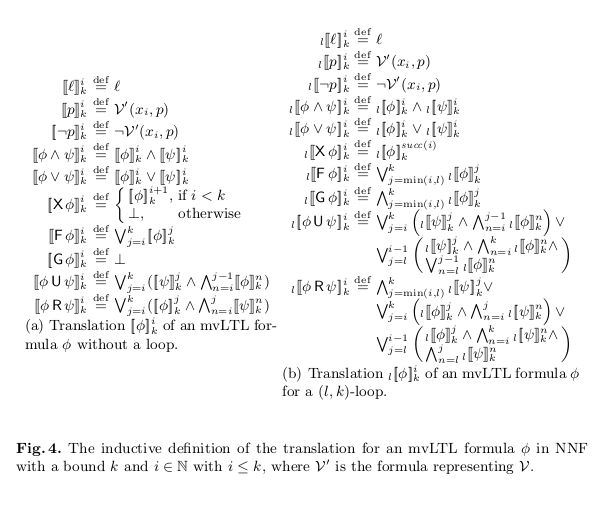# A Direct Algorithm for Multi-valued Bounded Model Checking### Resumo

Multi-valued Model Checking is an extension of classical, two-valued model checking with multi-valued logic. Multi-valuedness has been proved useful in expressing additional information such as incompleteness, uncertainty, and many others, but with the cost of time and space complexity. This paper addresses this problem, and proposes a new algorithm for Multi-valued Model Checking. While Chechik et al. have extended BDD-based Symbolic Model Checking algorithm to the multi-valued case, our algorithm extends Bounded Model Checking (BMC), which can generate a counterexample of minimum length efficiently (if any). A notable feature of our algorithm is that it directly generates conjunctive normal forms, and never reduces multi-valued formulas into many slices of two-valued formulas. To achieve this feature, we extend the BMC algorithm to the multi-valued case and also devise a new translation of multi-valued propositional formulas. Finally, we show experimental results and compare the performance of our algorithm with that of a reduction-based algorithm.

Publicação
6th International Symposium on Automated Technology for Verification and Analysis